# String

The string is one of the most fundamental objects in computer science. Strings are intimately familiar to us in the form of text and therefore it is no wonder that the theory of strings (which is to be distinguished from the field of theoretical physics known as "string theory") is one of the most extensively studied subdisciplines of computer science.

## Definitions

An alphabet, usually denoted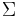$\Sigma$ is a finite nonempty set (whose size is denoted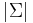$|\Sigma|$). It is often assumed that the alphabet is totally ordered, but this is not always necessary. (Since the alphabet is finite, we can always impose a total order when it would be useful to do so, such as when constructing a dictionary.) An element of this the alphabet is known as a character. Unless otherwise specified, we will always assume that two characters from the same alphabet can be compared for equality in constant time.

The set of$n$-tuples of$\Sigma$ is denoted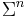$\Sigma^n$. A string of length$n$ is an element of$\Sigma^n$. The set$\Sigma^*$ is defined$\Sigma^0 \cup \Sigma^1 \cup ...$; an element of$\Sigma^*$ is known simply as a string over$\Sigma$. The empty string, denoted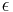$\epsilon$ or$\lambda$, is the unique element of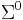$\Sigma^0$. The length of a string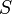$S$ is denoted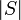$|S|$. (Note that the usual definition of "string" requires strings to have finite length, although arbitrarily long strings exist.)

It follows from the definition that all strings are sequences, but not all sequences are strings.

For ease of conceptualization, we shall usually assign a symbol, a graphical representation, to each character of the alphabet and, considering a string as a sequence of characters, render it as a sequence of symbols. We shall usually do so in boldface, but in this section we shall use italics to avoid confusion with terms being defined, hence, PEG. On other occasions we may choose to represent them with the ordered list notation, hence, [P,E,G]. (This form is useful when the symbols consist of more than one glyph, as can be the case when they are integers; see below.) We will often number the characters of strings, sometimes starting from zero, sometimes starting from one.

Examples of alphabets include:

• The binary alphabet, with exactly two characters (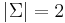$|\Sigma|=2$), usually denoted 0 and 1. In virtually all computers ever built, data are strings over the binary alphabet, and are known as bit strings.
• The Latin alphabet, with the characters a, A, b, B, ..., z, Z (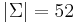$|\Sigma|=52$). English words may be considered strings over the Latin alphabet.
• The Unicode character set (the size of this alphabet depends somewhat on what we consider to be a valid Unicode character). Text files may be considered strings over this alphabet.
• The set of integers$\Sigma = \{0, 1, ..., N-1\}$ for some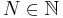$N \in \mathbb{N}$, for which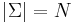$|\Sigma| = N$. Here the characters are also integers, and their corresponding symbols might have more than one glyph when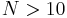$N > 10$. (Recall that the definition of character is broader than the common concept of the character as the smallest, indivisible element of writing.)
• The set of nitrogenous bases in DNA, {A, C, G, T}, with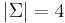$|\Sigma|=4$. Codons are considered members of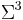$\Sigma^3$, and DNA sequences members of$\Sigma^*$.
• The set of universal proteinogenic amino acids, {A, C, D, E, F, G, H, I, K, L, M, N, P, Q, R, S, T, V, W, Y} ($|\Sigma|=20$). The primary structure of a protein is represented as a string over this alphabet. This and the preceding example are important alphabets in bioinformatics.

A substring of a string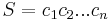$S = c_1 c_2 ... c_n$ is a string with the same alphabet as$S$ given by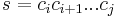$s = c_i c_{i+1} ... c_j$ for some$i, j \in \mathbb{N}$ with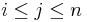$i \leq j \leq n$. Note that the empty string is considered a substring of all other strings. In other words, it is a series of (possibly zero) characters occurring consecutively in a string, taken in order. For example, the, in, here, and therein are all substrings of therein, as is$\epsilon$; however, tin is not (the characters are not consecutive in the original string), nor is rine (the characters do not occur in order in the original string). A prefix is a substring with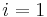$i = 1$, so that$\epsilon$, the, there, and therein are prefixes of therein. A suffix is a substring with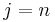$j = n$, so that$\epsilon$, in, rein, herein, and therein are suffixes of therein. We write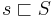$s \sqsubset S$ to indicate that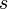$s$ is a prefix of$S$. Likewise the notation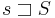$s \sqsupset S$ denotes that$s$ is a suffix of$S$. We say that$s$ is a proper prefix of$S$ when$s \sqsubset S$ and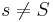$s \neq S$, with proper suffix defined analogously.

These examples may have given the misleading impression that strings which do not represent "valid" words, such as ther, are not valid strings. This is entirely untrue; ther is also a valid prefix of therein. The definition of a string says nothing about the validity or meaning of a string. A language is a (possibly empty, often infinite) subset of$\Sigma^*$; an element of a language is often called a word (although this term should be used with caution). Thus, while every element of$\Sigma^*$ is a valid string, not all are necessarily valid words in a language over$\Sigma^*$. For example, let$\Sigma$ be defined as the Latin alphabet and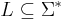$L \subseteq \Sigma^*$ be the language consisting of the representations of all valid English words. (Note that we have been careful to distinguish the words themselves from their representations as strings.) Then ther is certainly a valid string, being an element of$\Sigma^*$, but is not a valid word in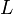$L$, since it does not represent an English word.

Thus, a string of length$N$ has$N+1$ prefixes,$N+1$ suffixes,$N$ proper prefixes,$N$ proper suffixes, and$1+N(N+1)/2$ substrings. (Here we consider two substrings to be distinct if they start or end at different indices, except that the empty string is counted only once; see also counting distinct substrings.)

Two strings with the same alphabet can be concatenated. To concatenate two strings is to link them together to give a new string which begins with the first and ends with the second with no overlap. Formally, the concatenation of strings$S$ and$T$ is denoted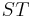$ST$ and has the following three properties:

•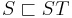$S \sqsubset ST$
•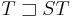$T \sqsupset ST$
•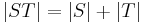$|ST| = |S|+|T|$

For example, concatenating there and in gives therein. So do concatenating the and rein, or$\epsilon$ and therein, or therein and$\epsilon$. Note that in general, concatenation is not commutative.

### Alphabet size

The size of the alphabet can affect the asymptotic complexity of string algorithms. In particular, if$N$ is the size of the input, then we can classify alphabets as follows:

• If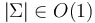$|\Sigma| \in O(1)$, that is, it is bounded above by a constant independent of$N$, then the alphabet is said to be constant.
• If the characters are integers bounded by$O(N^c)$ for some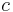$c$, then the alphabet is said to be integer. (This is equivalent to saying that the characters are integers with at most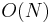$O(N)$ digits.) Note that this implies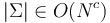$|\Sigma| \in O(N^c)$ and equivalently$\log|\Sigma| \in O(\log N)$.
• All alphabets are general, even when they are neither constant nor integer.

The dividing lines may seem arbitrary, but they are placed where they are because for many algorithms a better asymptotic complexity guarantee (in terms of$N$) may be achieved when the alphabet is constant rather than when it is integer, or when it is integer rather than when it is general. For example, assume that we want to construct a trie of a set of strings of total size$N$ using constant space. We need to store the links from each node (of which there may be up to$O(N)$) to its children. These links should be indexed by character, so that we can trace down a path of characters from root to leaf when looking for a word.

• When the alphabet is constant, we can simply store the child links of each node in an array and walk each link in constant time, and a search will take$O(N)$ time (because the trie's height cannot possibly be more than$N$), and furthermore the space complexity is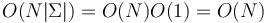$O(N|\Sigma|) = O(N)O(1) = O(N)$.
• When the alphabet is integer, this approach will not meet the linear space restriction. Instead, we can store the links at each node in a map (from character to pointer), which will allow us to perform the walk in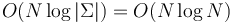$O(N\log|\Sigma|) = O(N\log N)$ time. The total extra space usage (that is, the total size of all the maps) is proportional to the total size of the maps, which, being equal to the total number of links, is$O(N)$.
• If the alphabet is general, and we do the same, then the time taken to walk down the tree will be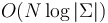$O(N \log |\Sigma|)$ again; but this is no longer bounded by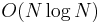$O(N \log N)$.

## Treatment in programming languages

In many programming languages, strings are superficially similar to arrays of characters, in that the syntax for accessing a string's characters usually match the syntax for accessing array elements. Some programming languages, such as C, go as far as to implement strings as arrays of characters. Other programming languages may have a separate library and separate set of functions for handling common string operations, such as:

• Insert one string into another at a specified position.
• Special case: Concatenate two strings (insert a string at the beginning or the end of another).
• Special case: Append a string or a character (which may be considered a string of length one) to the end of an existing string.
• Erase a substring of a given string at a specified position with a specified length.
• Special case: erasing from the end may be faster.
• Copy: Return a substring of a given string at a specified location with a specified length.
• Find the first occurrence of a given character in a given string, or find the first occurrence of a given string as a substring of another string.
• Compare two strings lexicographically.